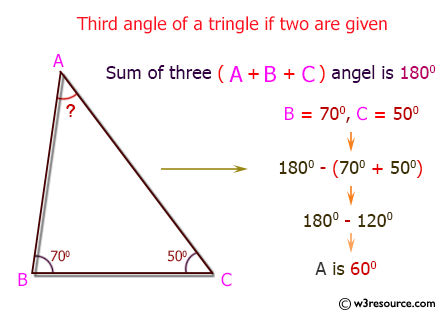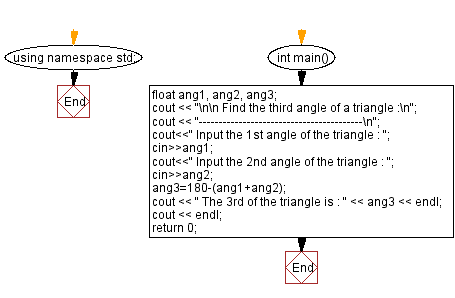﻿ C++ : Enter two angles of a triangle, find the third angle

# C++ Exercises: Enter two angles of a triangle and find the third angle

## C++ Basic: Exercise-52 with Solution

Write a C++ program to enter two angles of a triangle and find the third angle.

Pictorial Presentation:Sample Solution:

C++ Code :

``````#include<iostream>
using namespace std;

int main()
{
float ang1, ang2, ang3;
cout << "\n\n Find the third angle of a triangle :\n";
cout << "-----------------------------------------\n";
cout<<" Input the 1st angle of the triangle : ";
cin>>ang1;
cout<<" Input the 2nd angle of the triangle : ";
cin>>ang2;
ang3=180-(ang1+ang2);
cout << " The 3rd of the triangle is : " << ang3 << endl;
cout << endl;
return 0;
}
``````

Sample Output:

```Find the third angle of a triangle :
-----------------------------------------
Input the 1st angle of the triangle : 35
Input the 2nd angle of the triangle : 35
The 3rd of the triangle is : 110
```

Flowchart:C++ Code Editor:

What is the difficulty level of this exercise?

﻿

## C++ Programming: Tips of the Day

Why is there no std::stou?

The most pat answer would be that the C library has no corresponding "strtou", and the C++11 string functions are all just thinly veiled wrappers around the C library functions: The std::sto* functions mirror strto*, and the std::to_string functions use sprintf.

Ref: https://bit.ly/3wtz2qA

We are closing our Disqus commenting system for some maintenanace issues. You may write to us at reach[at]yahoo[dot]com or visit us at Facebook